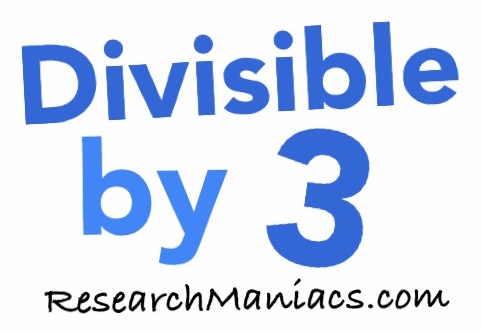Is 315 divisible by 3?Is 315 divisible by 3? In other words, if you divide 315 by 3, will you get a whole number with no remainder?

Of course, you could use a calculator to find out if 315 is divisible by 3, but what fun would that be?

To find out if 315 is divisible by 3, we will add up the numbers that make 315 as follows: 3 + 1 + 5 = 9

We know that if the sum of the numbers that make up 315 is divisible by 3, then 315 is divisible by 3.

Since the sum of the digits in 315 is divisible by 3, 315 is also divisible by 3. Thus, the answer to the question "Is 315 divisible by 3?" is as follows:

Yes

Note: If you divide 315 by 3, you get 105 which is a whole number.

Divisible by 3?
Do you need to check another number? Enter a number below to see if it is divisible by 3.

Is divisible by 3?

Is 316 divisible by 3?
Can you do it on your own now? If not, go here for the next number on our list.